# Predicting probabilities with PythonMost of the data science competitions I have been standard classification or regression predictions, I have so far come across two competition questions where the evaluation metric was the probability of a prediction rather than the actual prediction itself.

The sklearn library has the predict_proba() command that can be used to generate a two column array, the first column being the probability that the outcome will be 0 and the second being the probability that the outcome will be 1. The sum of each row of the two columns should also equal one.

In order to illustrate how probabilities can be predicted, I would like to use datasets taken from the Cross-sell Prediction on the Analytics Vidhya competition page, which can be found on the link below:- https://datahack.analyticsvidhya.com/contest/janatahack-cross-sell-prediction/#ProblemStatement

Below are excerpts from the Cross-sell Prediction Problem Statement:

“Your client is an Insurance company that has provided Health Insurance to its customers now they need your help in building a model to predict whether the policyholders (customers) from past year will also be interested in Vehicle Insurance provided by the company. Building a model to predict whether a customer would be interested in Vehicle Insurance is extremely helpful for the company because it can then accordingly plan its communication strategy to reach out to those customers and optimise its business model and revenue. In order to predict, whether the customer would be interested in Vehicle insurance, you have information about demographics (gender, age, region code type), Vehicles (Vehicle Age, Damage), Policy (Premium, sourcing channel) etc.”

The initial step in solving this conundrum is to load and read the libraries and files. Unfortunately the train and test sets are so large that they cannot be stored in a repository online (for free), so I had to save the files into my Google Drive account, where I am allotted 15 gigabytes of data for free: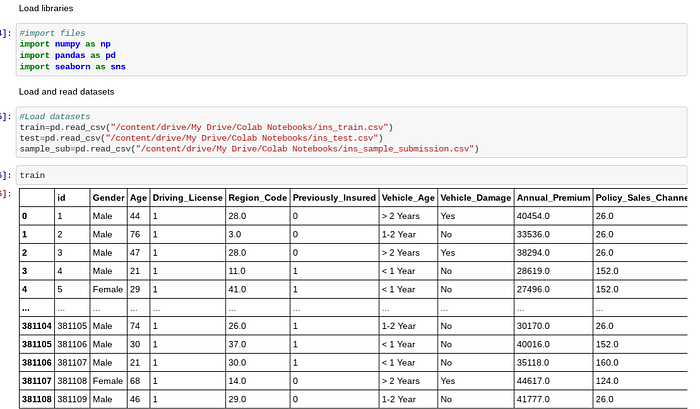I then checked for any null values and in this case there were none to impute: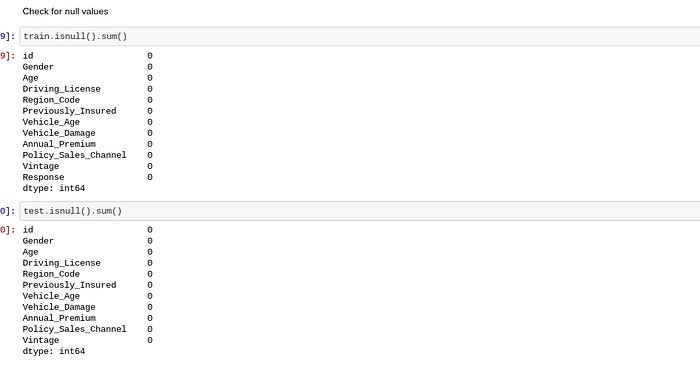I used the seaborn library to graphically represent the target variable. I then used Counter() to count the clause of 0 and 1. Both methods of interpretation revealed a class imbalance in favour of 0. This means the classes will need to be balanced to obtain the correct representation of 1's in the prediction: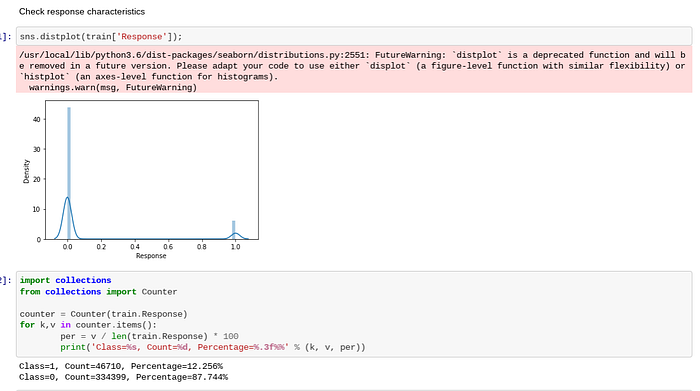I used LabelEncoder() to convert the object columns to numeric as a preprocessing measure. I then defined X, y and X_test. The train dataset was split using train_test_split() with 10% as the test set. Stratify is set to y because there is a class imbalance. The stratify parameter asks whether you want to retain the same proportion of classes in the train and test sets that are found in the entire original dataset. This setting ensures that the splitted data have at least some similarity between the train and test data: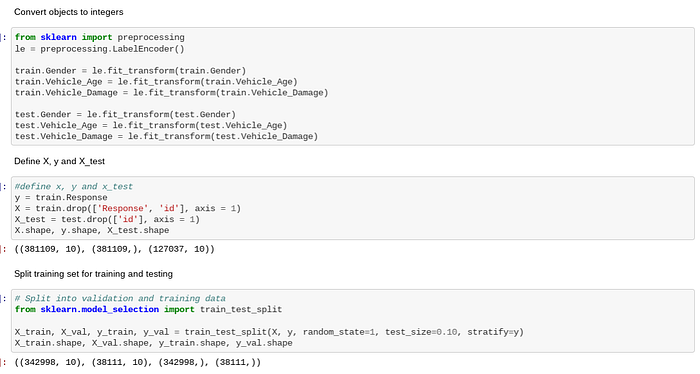I then defined class_weights, which would be used to fit X_train and y_train into the model and is used to balance the class weights to achieve a better accuracy. I selected XGBClassifier as the model because I have found XGBoost to give better accuracy than a lot of other models. XGBoost is an effective machine learning model, even on datasets where the class distribution is skewed.Although XGBoost is not part of the sklearn library, it is compatible and fits in well, as I have been able to use several sklearn functions with this model nonetheless. Using this methodology I was able to achieve 99.95% accuracy on the training set: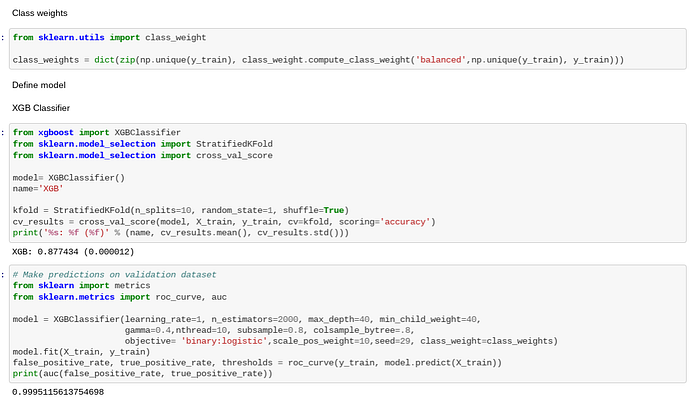When I predicted the validation set on the model I had fine tuned, I obtained an accuracy of 82.12%: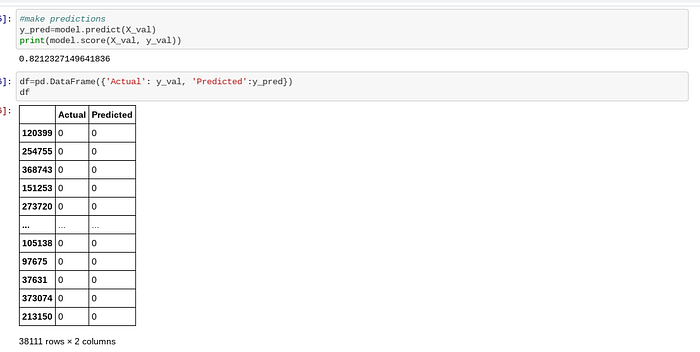I used the predict_proba() command to predict on the probability that a person would be interested in buying insurance: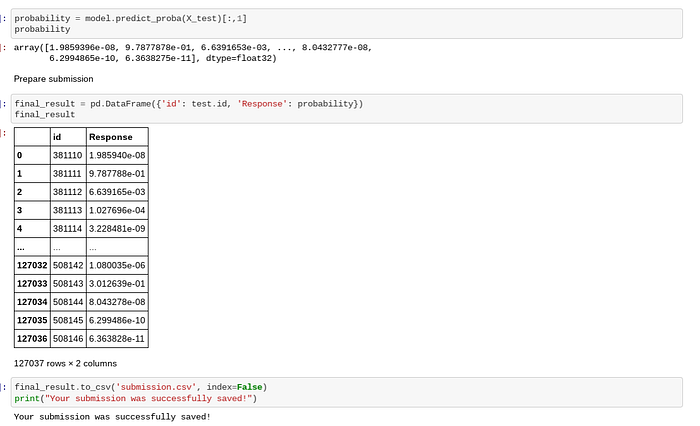When I submitted my prediction to Analytics Vidhya, I achieved a score of 80.77% which is not bad considering the highest score in this competition was 86.39%: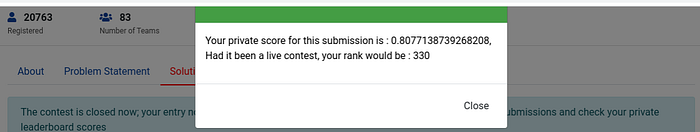The code used in this blog post can be found in its entirety on my GitHub account, found below:

https://github.com/TracyRenee61/Misc-Predictions/blob/main/AV_Insurance_XGBC.ipynb